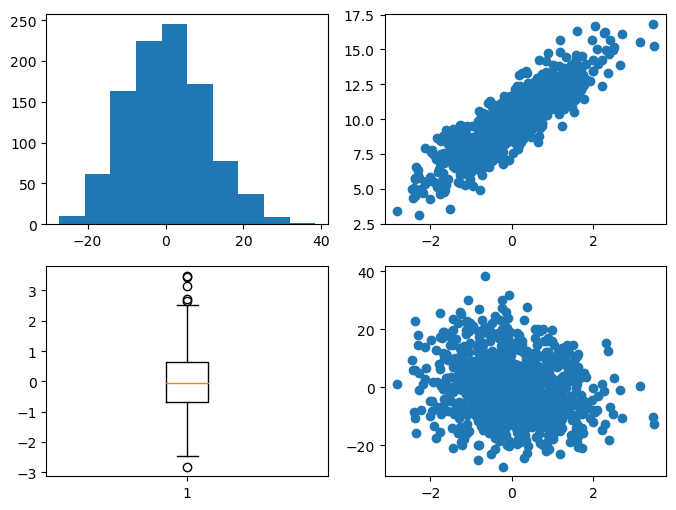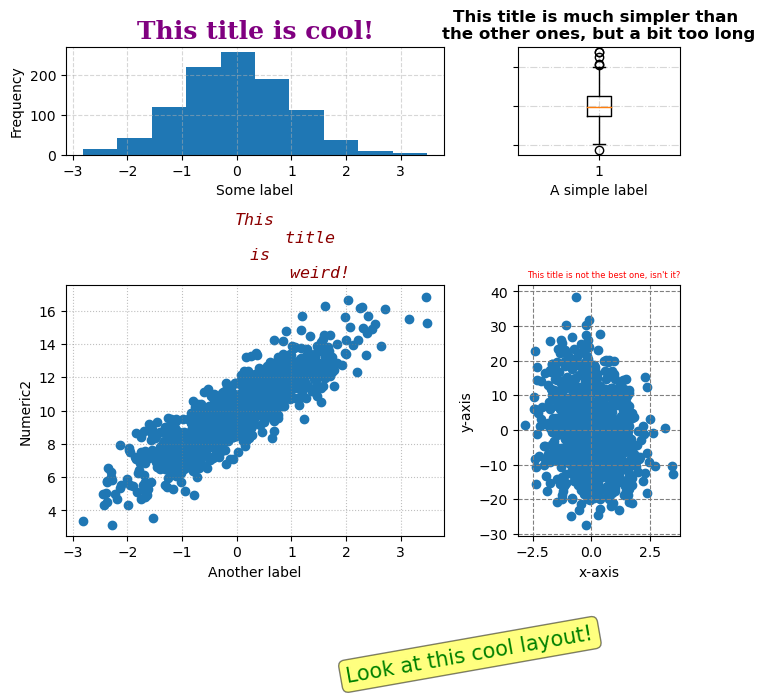## Libraries

First, you need to install the following librairies:

• matplotlib is used for plot creating the charts
• `numpy` is used to generate some data
• `pandas` is used to put the data into a dataframe
``````import matplotlib.pyplot as plt
import pandas as pd
import numpy as np``````

## Dataset

In order to be as exhaustive as possible, we need to create a dataset with enought variety.

• `Numeric1` and `Numeric2` are positively correlated variables, with `Numeric2` being calculated as Numeric1 plus some random noise.
• `Numeric3` is a negatively correlated variable to `Numeric1`.

We use the `random.normal()` function from `numpy` in order to create randomly distributed variables.

``````# Generate data for three numeric columns
sample_size = 1000

# Positively correlated variables
variable1 = np.random.normal(0, 1, sample_size)
variable2 = 2 * variable1 + np.random.normal(10, 1, sample_size)

# Negatively correlated variables
variable3 = -2 * variable1 + np.random.normal(0, 10, sample_size)

# Create a DataFrame
df = pd.DataFrame({
'Numeric1': variable1,
'Numeric2': variable2,
'Numeric3': variable3
})``````

## Initial graph

Here's the chart we're going to customize. It consists of a 2x2 frame with different types of graphics, but without any customization (all default argument).

``````# Create a 2x2 subplot layout
fig, axes = plt.subplots(nrows=2,
ncols=2,
figsize=(8, 6))

axes[0, 0].hist(df['Numeric3']) # Histogram in the upper left
axes[0, 1].scatter(df['Numeric1'], df['Numeric2']) # Scatter plot in the upper right
axes[1, 0].boxplot(df['Numeric1']) # Boxplot in the lower left
axes[1, 1].scatter(df['Numeric1'], df['Numeric3']) # Scatter plot in the lower right

# Show the plots
plt.show()``````## Customize the layout of the chart

Here are all the customization elements we are going to add:

• change the division of each subgraph on the global graph using the `add_gridspec()` function
• create a wide variety of titles: size, color, font, position, etc
• add different grids for each sub-graph using the `grid()` function
• add an annotation that says how cool our chart is using the `text()` function only
``````# Create a 2x2 subplot layout
fig = plt.figure(figsize=(7, 6))

# Define the grid layout
width_ratios=[7, 3],
height_ratios=[3, 7])

# Add annotation to the right
fig.text(0.5, -0.1, 'Look at this cool layout!',
fontsize=15, color='green', rotation=10,
verticalalignment='center',
bbox=dict(boxstyle='round',
facecolor='yellow',
alpha=0.5))

# Plot 1: Histogram in the upper left
ax1.hist(df['Numeric1'])
ax1.set_title('This title is cool!',
fontsize=18, fontweight='bold',
color='purple', fontfamily='serif')
ax1.grid(True, linestyle='--', alpha=0.5)

# Plot 2: Scatter plot in the lower left
ax2.scatter(df['Numeric1'], df['Numeric2'])
ax2.set_title('This\n           title\n is\n             weird!',
fontsize=12, fontstyle='italic',
color='darkred', fontfamily='monospace')
ax2.grid(True, linestyle=':', color='gray', alpha=0.5)

# Plot 3: Boxplot in the upper right
ax3.boxplot(df['Numeric1'])
ax3.set_title('This title is much simpler than \nthe other ones, but a bit too long',
fontsize=12, fontweight='bold',
color='black', fontfamily='sans-serif')
ax3.grid(True, linestyle='-.', alpha=0.5)

# Plot 4: Scatter plot in the lower right
ax4.scatter(df['Numeric1'], df['Numeric3'])
ax4.set_title("This title is not the best one, isn't it?",
fontsize=6, color='red',
loc='right')
ax4.grid(True, linestyle='--', color='gray', alpha=1)

ax1.set_xlabel('Some label')
ax1.set_ylabel('Frequency')
ax2.set_xlabel('Another label')
ax2.set_ylabel('Numeric2')
ax3.set_xlabel('A simple label')
ax3.set_yticklabels([])
ax4.set_xlabel('x-axis')
ax4.set_ylabel('y-axis')

plt.tight_layout()

# Show the plots
plt.show()``````## Going further

This post explains how to create a very customized layout in matplotlib.

For more examples of how to create or customize your plots with matplotlib, see the matplotlib section. You may also be interested in how to custom your fonts with matplotlib.

## Contact & Edit

👋 This document is a work by Yan Holtz. You can contribute on github, send me a feedback on twitter or subscribe to the newsletter to know when new examples are published! 🔥